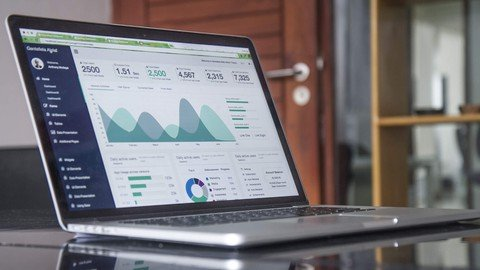﻿ Statistics and data analysis with Excel » GFXhome

» » Statistics and data analysis with Excel
Information of news
12-11-2021, 04:10

### Statistics and data analysis with Excel

Category: TutorialsStatistics and data analysis with Excel
Genre: eLearning | MP4 | Video: h264, 1280x720 | Audio: AAC, 44.1 KHz
Language: English | Size: 1.4 GB | Duration: 2h 32mHow to use Excel for statistics calculation and advanced data analysis

What you'll learn
Basic and advanced univariate statistics in Excel
Hypothesis tests (Student's t test, chi-square, F-test, Welch test), t-table and z-table
Confidence intervals for mean value and proportions
Correlation coefficient and linear regression forecast
Outlier identification
Database operations
Pivot tables

Description
In this practical course, we are going to focus on how to perform advanced statistical calculations and data analysis using Microsoft Excel.

Excel is a very used tool in several companies and has very powerful data analysis capabilities that can be used by data analysts and marketing experts. Even if you work with a lot of statistics you'd be surprised at how valuable Excel is for calculating hypothesis tests and the most common metrics you can calculate on a dataset. There are several basic and advanced functions you can use to get the best from your data and that's why Excel is a very useful tool for anybody who needs to crunch data and perform analyses of various kinds.

This course can be attended by both data analysts and marketing experts who need to work with data and surveys.

With this course, you are going to learn:

Univariate descriptive analysis (mean, standard deviation, skewness, quantiles, percentiles, IQR)

Advanced univariate analysis (outlier detection, rolling measures)

Confidence intervals

Linear correlation and regression forecasting

Hypothesis tests (Student's t-test, chi-square test, F-test, Welch test)

t tables and z tables

Database operations and conditional operations

Vertical lookup

Pivot tables

All the video lessons of this course start with a brief introduction and end with a practical example in Excel. All the Excel spreadsheets are attached to each lesson and can be downloaded.

Who this course is for:
Data analysts
Marketing experts
Data scientistsSite BBcode/HTML Code:
Dear visitor, you went to the site as unregistered user.